## 22 April 2015

### Hirschberg's algorithm explanation

Some time ago I needed to implement Hirschberg's algorithm but I couldn't find any good explanation of it. So here is mine attempt.

First of all: It's used to find the optimal sequence alignment. This alignment has to be measured somehow. As a metric we can use, for example, Levenshtein distance. Which means insertion, deletion and change have the same cost. This can be directly translated to computing list of all changes required to change one word into another.

Let's say we have a word L of size n and a word R of size m.

#### Needleman–Wunsch algorithm

First we need to understand Needleman–Wunsch algorithm. It's the easiest way to compute the alignment. The algorithm has just a few lines of code. Its time complexity is O(n*m), space complexity is also O(n*m). This is simple dynamic programming: having edge values we fill the array from top-left to bottom-right corner, choosing minimum (or maximum, depending on the metric. Let's say we are looking for the minimum edit distance). For L = bcd and R = abcde: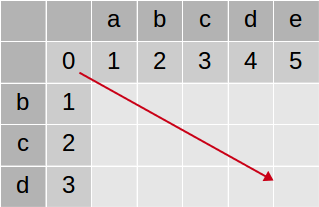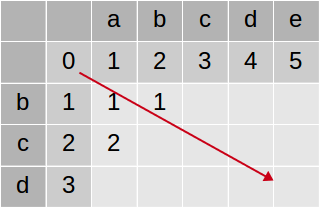After the whole array is filled we go backward (from bottom-right corner) and recreate the path.Each horizontal arrow represents an insertion, vertical - deletion and diagonal - match or replacement (depending if letters match or not). Therefore this array represents alignment:
```- b c d -
| | |
a b c d e
```
It also means that edit distance between bcd and abcde is 2. So we can change one into another with only 2 changes. But it's not all. We can get more information from this table. Last row contains all edit distances (D) between L and all prefixes of R:

D(bcd, abcde) = 2
D(bcd, abcd) = 1
D(bcd, abc) = 2
D(bcd, ab) = 3
D(bcd, a) = 3
D(bcd, ) = 3

If we are interested only in edit distance but not the whole alignment then we can reduce Needleman–Wunsch algorithm space complexity. The key observation is that we can fill the array horizontally (or vertically) so at any time we need only 2 adjacent rows of the array:Its space complexity is O(n) or O(m) (depending on the filling direction) and time complexity remains O(n*m). Let's call this version NW'. If we choose correct direction then last row also contains all edit distances (D) between L and all prefixes of R.

#### Hirschberg's algorithm

Hirschberg's algorithm lets us compute the whole alignment using time O(n*m) and space O(m+n). It uses NW' algorithm and divide and conquer approach:
1. if problem is trivial, compute it:
1. if n or m is 0 then there is n or m insertions or deletions
2. if n = 1 then there is m-1 insertions or deletions and 1 match or change
2. if problem is bigger, divide it into 2 smaller, independent problems
1. divide L in the middle. into 2 sublists L1, L2
2. find optimum division R = R1 R2
3. recursively find the alignment of L1 and R1
4. recursively find the alignment of L2 and R2
5. concatenate results
Of course point 2. needs better explanation. How can we divide big problem into smaller ones? Let's imagine some optimal alignment between L = a1...a27 and R = b1...b32:It means we can transform L into R in 15 operations (to be more precise: cost of transformation is 15). Obviously we can divide this alignment at any arbitrary position into 2 independent alignments, for example: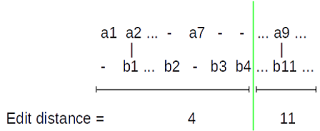Above edit distances is of course just an example. The point is, they must add up to the overall edit distance (15).

So now we have L = (a1...a7) (a8...a27) = L1 L2 and R = (b1...b4) (a8...a32) = R1 R2. It means we can transform L1 into R1 in 4 operations and L2 into R2 in 11 operations. We can concatenate those transformations to get the overall result. It means that if we somehow know the right division we'll be able to compute the alignment of L with R by computing alignments of L1 with R1 and L2 with R2 and simply concatenating results. So it will be possible to split big problem recursively until we reach trivial cases. For example (from wikipedia) to align AGTACGCA and TATGC we could do:
```(AGTACGCA,TATGC)
/              \
(AGTA,TA)            (CGCA,TGC)
/     \              /      \
(AG,)   (TA,TA)      (CG,TG)  (CA,C)
/   \        /   \
(T,T) (A,A)  (C,T) (G,G)
```

But if we have L divided into L1 and L2 how do we know where to divide R? Let's say we want to align (bcdce, abcde)Firstly, let's arbitrary divide L in the middle. So we have two new lists L1 and L2.So where is the correct division of R? Well, we just need to ensure that edit distance (L1, R1) + edit distance (L2, R2) will not be larger than the overall edit distance (L, R). That is, we will use the shortest possible transformation (or alignment). How to ensure that? Well, some of the possible divisions are the optimal ones. So just check all of them and pick the one with the smallest sum of edit distances.
Ok, but how to quickly compute edit distances of L1 with all possible R1 and L2 with all possible R2? Here NW' algorithm can help us. It's straightforward to run for the upper half: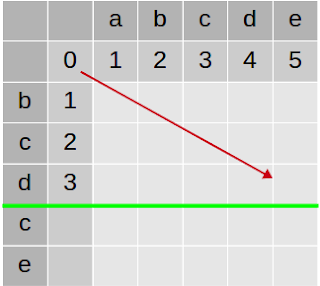After NW' run is completed we have edit distances between L1 and all possible R1:But what about L2 and all possible R2? Again NW' can help, we just need to run it backward. After all edit distance is the same if we look at both words forward or backward, right?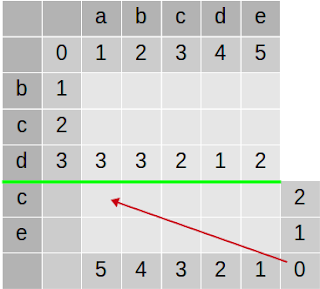After NW' run is completed we have edit distances between L2 and all possible R2:Now, we need to choose the partition of R. Let's say we choose to divide R = abcde into R1 = empty and R2 = abcde:Now we would have to align L1 = bcd with R1 = empty and L2 = ce with R2 = abcde. What would be the edit distances?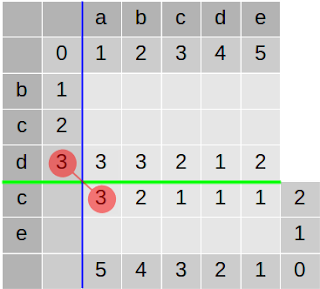It means we can transform L1 (bcd) into R1 (empty) in 3 operations and L2 (ce) into R2 (abcde) in 3 operations. So choosing this division we would transform L into R in 6 operations. In other words cost of alignment of L and R with this division would be 6. Can we do better? What if we place the dividing line elsewhere?Now we would align L1 (bcd) with R1 (a) with cost 3 and L2 (ce) with R2 (bcde) with cost 2. Overall alignment cost (edit distance) would be 3 + 2 = 5. And so on. The last possible position is:Which is aligning L1 (bcd) with R1 (abcde) with cost 2 and L2 (ce) with R2 (empty) with cost 2. Overall cost (distance) is 2+2 = 4. Obviously the smallest overall edit distance is: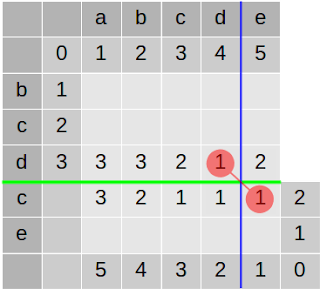So this is the division we were looking for! Now we have 2 independent subproblems: align L1 = bcd with R1 = abcd and L2 = ce with R2 = e. Let's look at the array, it's divided into 4 parts and now we are only interested in top-left and bottom-right part. We will never ever need to look at top-right and bottom-left part. So the overall problem has been reduced by half: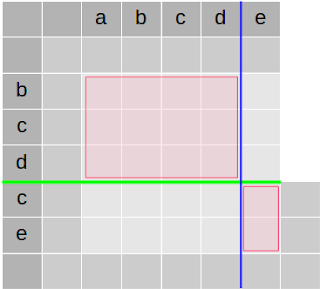That's it. Rest is just basic but a bit tedious math on subarray boundaries and debugging off-by-one errors.

#### Complexity

space: We need to store L and R. Each NW' run uses only 2 rows of size n. Each time we divide L by 2 so the recursion depth is O(log n), Therefore total used space is O(n+m)

time: At each step we run NW' on the whole L x R array. Later 2 subproblems are created of the total size of the half of the original array. So number of operations is: (1 + 1/2 + 1/4 + ...) * (n*m) = O(n*m)